# Introduction to MATLAB for Python Users

Today’s blog is written by Jennifer Rebbin, Jennifer is an Application Support Engineer with the Engineering Development Group at MathWorks. In this blog, she shares some important concepts that will help get you up to speed with using MATLAB as a Python user. Jennifer, over to you..

You have some Python experience – maybe from a programming or data science class, or maybe from fun side projects. And now you are digging into your engineering career or classes and need to use MATLAB. There are a lot of similarities between MATLAB and Python, so your Python experience should be a good head start for learning MATLAB. This blog post examines the similarities and differences between MATLAB and Python and provides syntax examples for applying general programming concepts in MATLAB.

# Learn the basics of MATLAB programming

The basic data structure in MATLAB is a matrix, while Python treats everything as a general object. In fact, MATLAB is an abbreviation for “matrix laboratory”– this difference is an integral part of what defines MATLAB.

Let’s learn about MATLAB programming with an example using a MATLAB dataset popcornThe data is from a study of popcorn brands and popper types (Hogg 1987). The columns of the matrix are popcorn kernel brands, Gourmet, National, and Generic, respectively. The first three rows correspond to batches using an oil popper, and the last three rows correspond to batches using an air popper. The response values are the yield in cups of popped popcorn.

We can load the data in the Command Window panel of the MATLAB Desktop.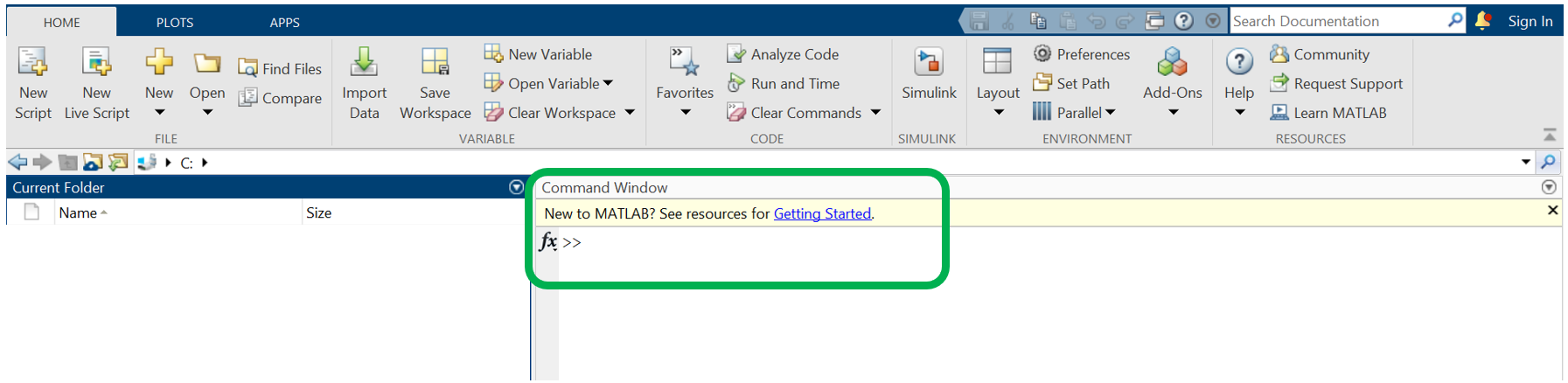load 'popcorn';
popcorn
 popcorn =
5.5     4.5    3.5
5.5     4.5     4
6       4      3
6.5      5      4
7       5      5
7       5     4.5


Now that we have our data, we want to perform a simple calculation to determine the ratio of popped popcorn between the Gourmet and National brands. Since we don’t specify an  output variable, MATLAB uses the variable ans to store our calculation results.

popcorn(:,1)./popcorn(:,2)
 ans =
1.22
1.22
1.5
1.3
1.27
1.4


MATLAB was created for linear algebra so operators such as the division operator “/” produce conventional matrix operations, while some Python libraries perform operations element-by-element. To perform element-wise operations in MATLAB, a period is placed before the operator, as in “./.

To learn more about common MATLAB syntax and its functionality, reference the table below or this comprehensive guide.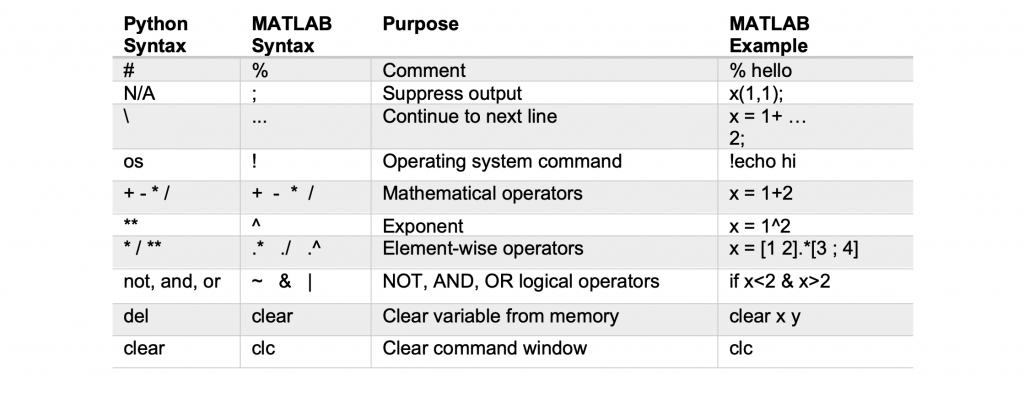# Index data and function outputs

Next, let’s learn about indexing into data and function outputs. In MATLAB, the beginning element of an array has an index of 1, unlike in Python where the beginning element has an index of 0.

MATLAB uses N-D indexing, or providing a specific index value for each array dimension (such as a row number and a column number). It’s important to note that indices in Python are left inclusive and right exclusive but MATLAB is inclusive on both ends.

popcorn
 popcorn =
5.5    4.5    3.5
5.5    4.5     4
6      4      3
6.5     5      4
7      5      5
7      5     4.5

 % View batches with popper type air with N-D indexing
popcorn(4:end,:)
 ans =
6.5     5     4
7     5.5    5
7      5    4.5

 % View batches with popcorn brands Gourmet and Generic with N-D indexing
popcorn(:,[1 3])
 ans =
5.5    3.5
5.5     4
6      3
6.5     4
7      5
7     4.5


MATLAB also uses linear indexing where a single subscript traverses down each column of an array in order, or logical indexing where MATLAB extracts matrix elements corresponding to non-zero elements of a logical array produced by using an operator like “>” in indexing. Find more information about these indexing strategies in this article.

 % View the ninth batch with linear indexing
popcorn(9)
 ans = 4
 % View batches producing more than 6 cups of popcorn with logical indexing
popcorn(popcorn>6)
 ans =
6.5
7
7


To expand an array, in Python you could append the item. In MATLAB, array size increases automatically to accommodate new elements outside index bounds which allows us to quickly manipulate arrays. If information is missing, MATLAB pads the matrix with zeros to keep it rectangular. Let’s add a new column for a fourth popper brand to be tested.

popcorn(1,4) = 0
 popcorn =
5.5    4.5    3.5    0
5.5    4.5     4     0
6      4      3     0
6.5     5      4     0
7     5.5     5     0
7      5     4.5    0


Now that we know how to expand an array in MATLAB, let’s revert to the original matrix. To remove the column we just added, simply execute the following.

popcorn(:,4) = [];

MATLAB and Python incorporate slightly different referencing schemes. Check out the table below to observe additional uses of MATLAB referencing syntax.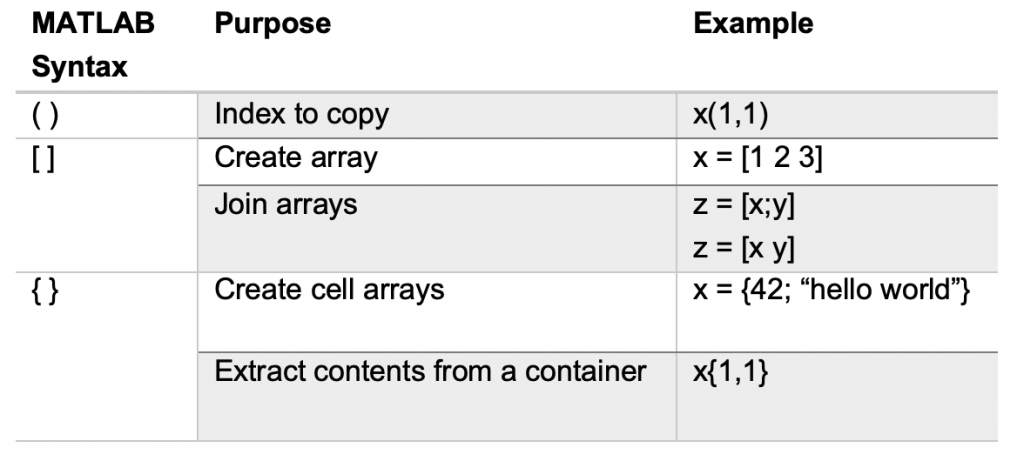# Write and use MATLAB functions

Now that we understand basic MATLAB programming syntax and indexing, let’s explore MATLAB built-in function mean and find the average popcorn yield for each popper brand. When calling built-in functions in MATLAB, we won’t need to reference a package name.

brandAvg = mean(popcorn)
 brandAvg =
6.25    4.75    4

Let’s create a user-defined function popperTypeAvg in a new Function file to compare the yield of oil and air popper types. MATLAB uses function and end, while Python uses defWe will use one input argument and two output arguments to bring variables from the function workspace to our MATLAB base workspace.

 % Saved as function file on MATLAB path called popperTypeAvg.m (file and function names must match)
function [firstHalfAvg, secondHalfAvg] = popperTypeAvg(data)
rows1to3 = mean(data(1:3,:),'all')
rows4to6 = mean(data(4:6,:),'all')
end


Now let’s call our function popperTypeAvg, declaring the input as our dataset popcorn and the outputs as variables oilAvg and airAvg.

[oilAvg,airAvg] = popperTypeAvg(popcorn)
  oilAvg = 4.5
airAvg = 5.5

In addition to creating a function definition file, there are other ways to define functions in MATLAB including local functions at the end of script filesnested functions located within another function, and anonymous functions defined in an executable statement.

# Visualize data

Let’s visualize our data and get a better idea of which brand yields the most popcorn. We can adjust figure properties within the function call using name-value pairs, or with separate commands.

bar(popcorn,'BarWidth',.75)
brandNames = ["Gourmet","National","Generic"];
legend(brandNames,'Location','bestoutside')
title('Popcorn Yield')
xlabel('Batch Number')
ylabel('Cups of Popped Popcorn')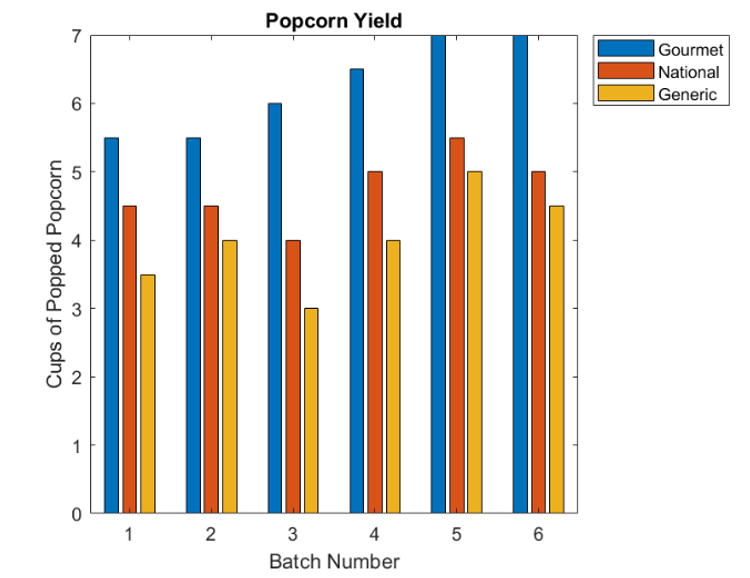If we want to create one figure with three plots (one for each popcorn popper brand), we can use the MATLAB function tiledlayout and specify the number of rows and columns as input arguments.

% To obtain the number of columns of an array, we can specify the second dimension in size(A,dim)
tiledlayout(1,size(popcorn,2))
for i = 1:size(popcorn,2)
nexttile
bar(popcorn(:,i))
title(brandNames(i))
xlabel('Batch Number')
ylabel('Cups of Popped Popcorn')
end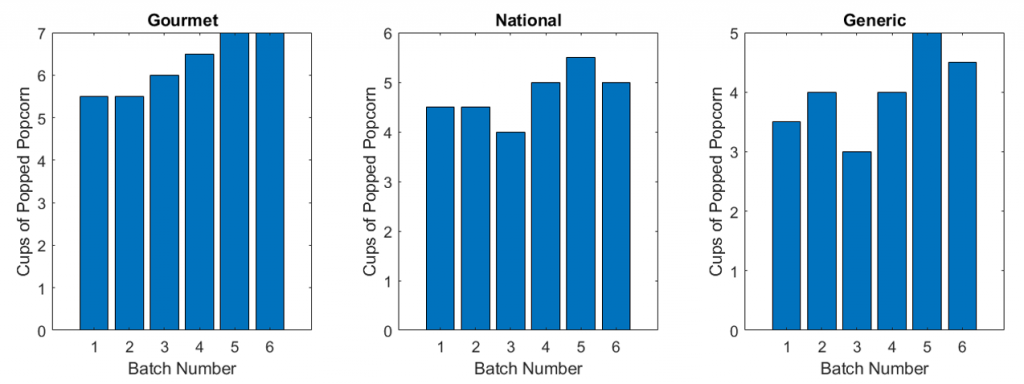# Understand data types

Unlike some other languages, neither Python nor MATLAB require declaring a variable or variable typea variable is created the moment it is assigned value.

While in Python it is easy to accidentally create ints and special constructors may be necessary to create numeric arrays, MATLAB’s default numeric class is double-precision floating point. In MATLAB, “1” and “1.” are always equal. Doubles can be complex and accept most mathematical operations. To view a variable’s data type, you could use type in Python. In MATLAB we can use the whos function, or view the variable data type in the MATLAB Workspace Browser.

whos 'popcorn'
Name     Size   Bytes   Class   Attributes
popcorn  6x3     144    double


MATLAB has built-in functions for converting data types. Let’s explore one of these functions array2table to create a table and label the columns of our popcorn dataset.

brandNames = ["Gourmet","National","Generic"];
array2table(popcorn,'VariableNames',brandNames)
 ans =
6x3 table
Gourmet    National    Generic
_______    ________    ________
5.5        4.5         3.5
5.5        4.5          4
6          4           3
6.5         5           4
7         5.5          5
7          5          4.5


In MATLAB, the logical data type can have value “0” for false or “1 for true. Let’s explore this behavior by utilizing logical indexing and a conditional statement to determine if the Gourmet brand kernels produced the largest average batch.

brandAvg = mean(popcorn)
(brandAvg(1)>brandAvg(2))&&(brandAvg(1)>brandAvg(3))
brandAvg =
6.25   4.75   4

ans =
logical
1

Other data type options such as character, date, categorical array, structure, and cell array can be explored in the MATLAB data types documentation or in the table below.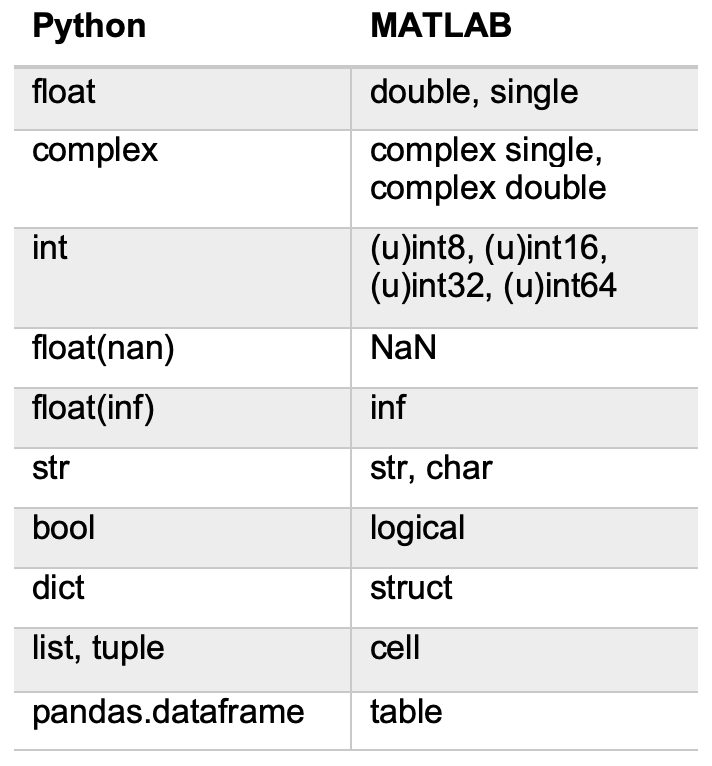# Implement looping behavior

In programming, loops repeat a sequence until a specified condition is met. Often, loops resize arrays and require MATLAB to continuously look for larger contiguous blocks of memory. One trick to improve code execution time when using loops and conditional statements in MATLAB is pre-allocation, or establishing the maximum amount of space required for the array. To pre-allocate an array, create an array with the intended resulting size and then replace the elements, rather than changing the array size with each iteration.  Let’s find the kernel brand with the highest yield for each batch.

%First, pre-allocate the bestBrand array and change the data type to categorical
bestBrand = zeros(6,1);
bestBrand = categorical(bestBrand);
% For each row, check which column contains the maximum yield
for i = 1:6
if popcorn(i,1) == max(popcorn(i,:))
bestBrand(i) = 'Gourmet';
end
if popcorn(i,2) == max(popcorn(i,:))
bestBrand(i) = 'National';
end
if popcorn(i,3) == max(popcorn(i,:))
bestBrand(i) = 'Generic';
end
end
bestBrand
bestBrand =
6x1 categorical array

Gourmet
Gourmet
Gourmet
Gourmet
Gourmet
Gourmet

From this analysis, we see the Gourmet brand produced the most popcorn in all six batchesWhile this method provided the desired resultthe code is lengthy and takes a long time to execute. How could we find the best brand more efficiently?

MATLAB often doesn’t require writing loops as it is “vectorized”. MATLAB is optimized for matriand vector operationsso writing vectorized code can improve code readability, speed, and memory. We can vectorize our loop by calling max on the entire popcorn array rather than one row at a time. The max function returns multiple outputs, so we will capture these in square brackets.

% To return the max of each row as a column vector, we specify the second dimension in max(A,[],dim)
[maxVal,index] = max(popcorn,[],2);
brandNames(index)
ans =
1x6 string array
"Gourmet"  "Gourmet"  "Gourmet"  "Gourmet"  "Gourmet"  "Gourmet"

Check out the table below for more looping behaviors and conditional statements to incorporate in MATLAB.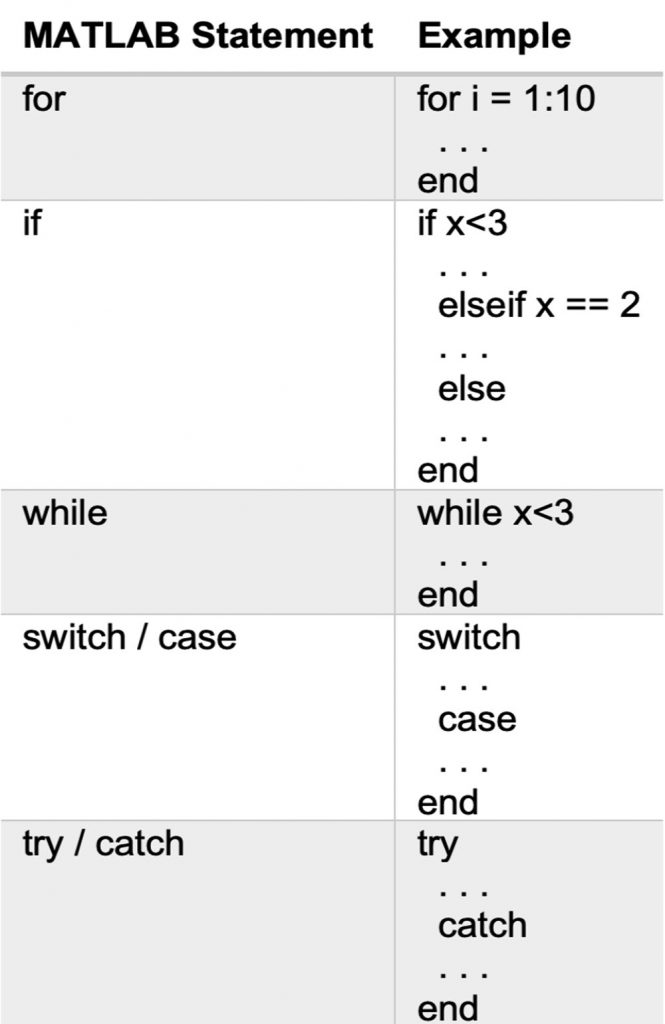# Define a custom class

Wcan use object-oriented programming when building an application to group parameters (properties) and functions (methods) into one class. While Python classes can be defined anywhere, MATLAB classes are created in a class definition file. MATLAB classes can be useful to have more control over what can and cannot be done to an object, such as defining a property as “constant” to disable modification. Check out an example utilizing sensor array data or follow the class framework below.

 % Saved as file MyClass.m, class name must match file name
classdef MyClass
properties
prop
end
methods
function obj = MyClass(val)
end
end
end 

The above class framework shows the MATLAB default copybywrite value class, as opposed to a copy-by-reference class. Check out this page outlining the differences between these two class types.

# Closing thoughts

We hope this information paints a picture of the functional similarities and differences between the Python and MATLAB languages. Hopefully you are finding MATLAB easy to learn and can use this information to get started. For more introductory resources, check out our two hour MATLAB Onramp tutorial, read Get Started with MATLAB, or dive into the MathWorks documentation and articles linked throughout this blog. Be sure to comment below or use your preferred social media platform to share what you’re working on and ask any questions.

|